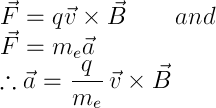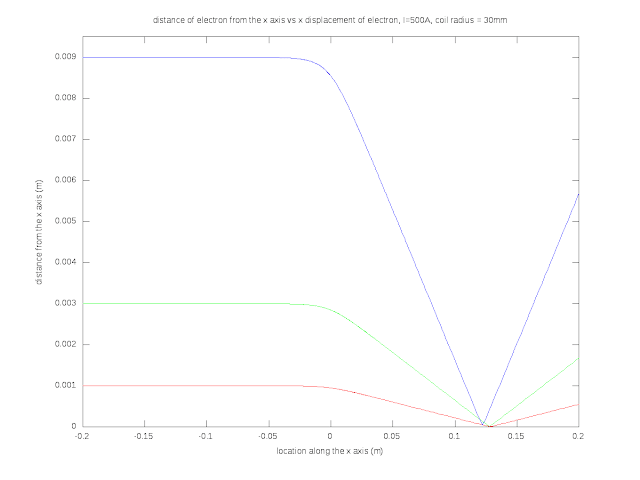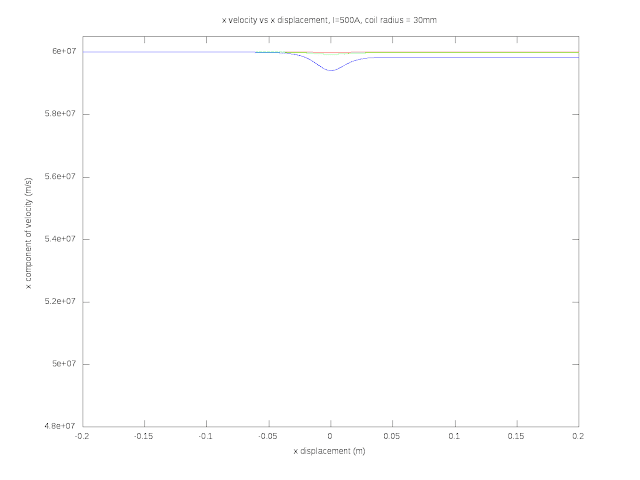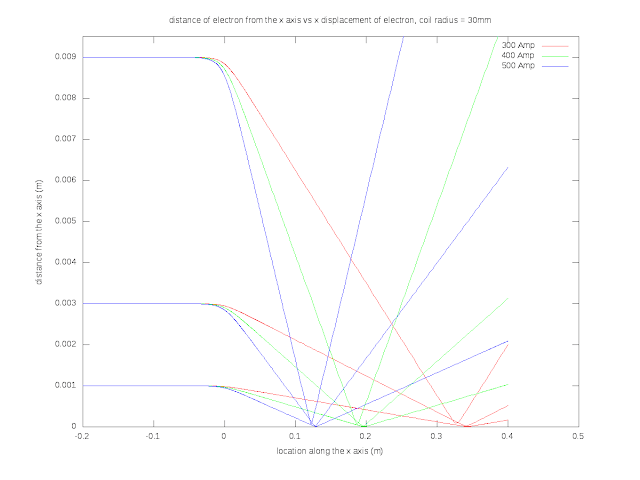## Friday, April 27, 2012

### I hope you find this blog enjoyable and helpful. Support it with a small donation.Solenoid field intensity

A while back I explained how cathode ray tubes (CRT) work as part of another blog post, and I understood all of it except for one thing, how on earth did the magnetic focussing coil work.  So I made it my goal to simulate the physics and see what came out of it.  GNU Octave turned out to be handy for this as I already knew MATLAB.

To model the problem an electron is fired towards a current loop parallel to the loop's axis and the trajectory is observed.  The loop is in the y-z plane centred at the origin with the electrons fired in the positive x direction.  First things first, this is a basic simulation, it models the electron as a classical particle with a negative charge.  I'm not taking relativity into account, even though the speed of the electrons in the simulation are 20% of the speed of light after being accelerated through 10 kV, so relativistic effects should be starting to become apparent.  The simulation is for a single electron, so there are no repulsive forces between electrons in the beam causing blooming.  Radiation given off by accelerating charges is not taken into account.

I am going to use a modified version of the formula for the magnetic field of a current loop that I calculated in a previous post.  These equations are for a current loop in the x-y plane and will need to be rotated for a loop in the y-z plane.Magnetic field equations

These equations along with an ODE solver in Octave are used to calculate the trajectory and plot some graphs and analyse the results.  I won't explain the code it's pretty simple.  You start with the initial position and velocity for an electron, you put that into the solver along with the equations describing the forces acting on the electron and you get an answer.  The comments in the code should go a long way to describing how it works.  Most of it is just for displaying the graphs, so although it looks hard it really isn't.  The files are available here.

The magnetic field generated by the current loop acts upon the electron with the Lorentz force as shown below.Lorentz force
By combing that with Newtons second law of motion we obtain the formula for acceleration.electron acceleration
This shows that the acceleration of the electron is proportional to the cross product of the velocity and the magnetic field which means it's accelerated perpendicular to its velocity and will tend to travel in arcs.  You can get a conceptual idea of how the coil focuses electrons with a thought experiment.  Firstly the electron comes in parallel to the coil axis and experiences no force from the component of the magnetic field parallel to the axis.  The component of the field perpendicular to the axis accelerates it tangentially and gives it a velocity perpendicular to the axis.  This velocity now causes a radial force moving the electron towards the axis focussing it.

A real life coil used to focus the beam would have many turns on it, but to start with I am going to simulate it as if all the turns are in the same place.  So instead of 1000 turns carrying 500mA, I can simulate one turn with 500A.

The parameters for the simulation were chosen to be close to reality, but I wasn't too concerned with the electron velocity, or accurate dimensions for the coil as this is just to investigate the lensing effect.  So for the first simulation I selected 500 A for the coil current and a radius of 3cm.  Three particles starting 1mm, 3mm, and 9mm from the coil axis are simulated.

It may be useful to start with a graphical representation of the field.Cross section of the field through the coil axisCross section of the field just above the plane of the coilEvidence of focussing

In the graph above we can see that the three particles are are forced towards the axis near the coil at x equals 0, and are focussed near the point x equals 0.15 meters.  There is evidence of aberration at the focal point just like an optical lens, but at one point all beams come within approximately 0.2 mm of the coil axis.x velocity through the simulation
An interesting property of an electron moving in a magnetic field is that it doesn't change speed.  The velocity will vary but the speed will remain constant, this means it can turn but not speed up or down.  Therefore the magnitude of the velocity vector should remain constant.  For a speed change, work must be done on, or done by the electron, and if we have a look at the definition of work it will become obvious why the speed stays constant.

As the force applied to the electron is always perpendicular to the velocity, no work can be done on it or by it.  This means the electron's kinetic energy remains constant.  The plot above shows that after the interaction with the lens, the electron loses velocity in the x direction, meaning it now has velocity in the plane of the coil.velocity magnitude

One way to verify our simulation is to check the velocity magnitude.  It should not change throughout the simulation.  The graph above does show a slight variance but if you look at the scale on the y axis it is incredibly small, too small to render useful numbers.  This error is well within what I would expect for general inaccuracies in a numerical simulation.  If there were problems like instability in the equation describing the field, or problem in the code I would expect a much larger effect.  This builds confidence in the results.Electrons curving towards the axis
As predicted, the electron will move in an arc and move towards the axis.  I did expect them to spiral afterwards though.  Once through the lens they are thrown off in different directions in essentially a straight path.electrons converging at the focal point
From the above graphs we get an insight into how the lensing effect works.  It's not exactly as I expected but it's close.Focus points for different coil currents

One property of the focussing coil in a CRT that's essential is the ability for it to adjust its focus.  As the beam scans along the front of the tube, the length of the path the electrons travel changes.  The graph above shows what happens as the current varies.  A coil current of 500 Amps focusses at around 12 cm, 400 Amps focusses at around 19cm, and 300 Amps focuses at around 33cm.

This was a fun exercise that illustrates a complex problem with a few basic equations.  I plan to do another couple of posts based on this simulation using different parameters and configurations.  It may seem odd to look into how CRT's work since they are all but extinct, but there are plenty of other applications like electron microscopes that use the same technology, and with a bit of tweaking the code could easily model gravitational problems.